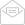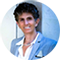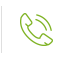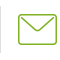05.07.2009

1 tcf of gas is equal to 28.3 bcm

1 bcm of natural gas is equal to 35.7 trillion British Thermal Units (or 35,714,286 MMBtu as per the EIA and used by the PUA). 1 bcm is equal to 35.3147 billion cubic feet (bcf), is equal to 0.83 million tons oil equivalent (mmtoe) (or 900,000 tons according to the Israeli ministry of energy conversion rate), is equal to 6.1 million barrels oil equivalent (mmboe) (or 6.6 according to the conversion rate used by the Israeli Ministry of Energy), is equal to 17.23 thousand barrels of oil, is equal to 96.7 million standard cubic feet, is equal to 0.73 million tons of LNG.

1 million ton of oil equivalent of natural gas = 1.11 bcm of natural gas

1 bcm of gas is equal to 678,000 tons of gas.

1 bcm of gas or 35.7  million mmbtu of gas = to find the equivalent in gasoil one has divide by 43.218 to get amount in tons (then find the price of gasoil in tons and you get the equivalent price of gasoil per 1 bcm of natural gas) or for fuel oil/mazut divide by 41.2. (one ton of mazut/fuel oil is about 40 mmbtu)

To convert CM3 and Sm3 =

Divide 36.32995129 for ‘000 Sm3 to MMBtu
Divide 35.7149 for ‘000 Cm3 to MMBtu (for Turkish cm3)

For regular cm3 multiply by 35.31 to get to mcf (then depending on the conversion between mcf and mmbtu

1,000 cm (kscm or mcm or thousand cubic meters) is 36.2 MMBtu.  To convert a price from \$1,000 cm to \$/MMBtu = divide the \$mcm  by 36.2 to get the price in \$/MMBtu

1 MTPA of LNG = 48.7 Bcf/y.

One million tons of LNG is equal to between 1.38 – 1.41 bcm of gas.

1 tcf = 172 mmboe

6.1 mcf = 1 bbl (BOEs may be misleading, particularly if used in isolation. A BOE conversion ratio of 6 McF: 1 bbl is based on an energy equivalency conversion method primarily applicable at the burner tip and does not represent value equivalency at the well head.

1 TJ =

1 TJ =947.817 MMBTU

1 MMBtu = 27.8 kscm/mcm.  so if 1 MMBtu is \$7, that is 27.8 mcm at \$7, so to reach the price of 1,000 kscm take \$7 divided by 27.8 multiplied by 1,000 = 251

You can convert prices from one basis to another:
\$ per Ccf divided by 1.032 = \$ per therm
\$ per therm multiplied by 1.032 = \$ per Ccf
\$ per Mcf divided by 1.032 = \$ per MMBtu
\$ per Mcf divided by 10.32 = \$ per therm
\$ per MMBtu multiplied by 1.032 = \$ per Mcf
\$ per therm multiplied by 10.32 = \$ per Mcf

If you have the price of natural gas in tons and want to convert it to the price per mmbtu: 1 bcm of gas is equal to 678,500 tons of gas. 1 bcm is equal to 36,000,000 mmbtu and one ton of natural gas is equal to 52.4 mmbtu (as per IEC data book). Thus, you take the price per ton (e.g. 815 NIS per ton) and divide this by 52.4 to get the price per mmbtu in shekels and then convert it to dollars.

To calculate the price in mmbtu if you have the price in bcm. For each 1 bcm divide the total sale price by 1 billion and multiply by 28.317. Example: If 1 bcm of gas costs \$250,000,000. Divide this figure by 1,000,000,000 and multiply by 28.317. This assumes the gas has CV of 1,000 btu/cu ft. At the assumed CV of 1,000 btu/ft3 there are 35,315 btu per m3. This means there are 28.317 m3 per mmbtu. Obviously the price would go up or down if the CV was higher or lower than the assumed 1,000 btu per ft3.

To convert from BCM to mmbtu: multiply the bcm by 1 billion (e9) and divide by 28.317 (e.g. 50 bcm multiplied by 1 billion divided by 28.317 = 1,785,714,286 mmbtu

To convert the price of 1 mmbtu of gas into the price for 1 bcm, multiply the price by 36.7 million, so that if for instance natural gas is priced at \$5 mmbtu then the value for each 1 bcm is \$183.5 million.

To convert a quantity of gas of 1 bcm per year into mmscfd (if gas has CV of 1,000):
Multiply 1 bcm * 35.315 and divide by 365
To get amount of mmscf per hour divide by 24
If want to know how much a station uses per year multiply by number of annual hours (e.g. 7,000)

To convert quantities from mmbtu to bcm multiply the mmbtu by 28 and divide by 1,000,000,000. For example 7660000 mmbtu per annum of natural gas consumption is equal to 0.21448 bcm.

To convert from billion cubic feet to billion cubic meters divide the bcf figure by 35.3. To convert from bcm to bcf * by 35.3.

To obtain the prices of an mmbtu of gas if one has the price in a thousand cubic meters, one needs to divide the price by 35.315.

To link the price of natural gas to that of oil (e.g. Brent) one needs to divide the price by 5.8, so that if for instance Brent costs \$80 a barrel, the equivalent in mmbtu of natural gas would be \$13.79. Since natural gas is often sold at a discounted percentage of oil (potentially 50% thereof), a formula could be something like 50% * (brent/5.8).

To convert to the price in tons equivalent one would need to multiply by 7 so that \$80 a barrel would be equal to \$420 ton.

To convert the price of gas from \$/MW to \$/MMBtu divide by 3.408

HFO – to convert from bbls of Brent to tons of HFO multiply the Brent by 7.54. If you want to then convert the price from Brent to. To go from \$/tons of HFO to mmbtu in HFO (divide the HFO \$/tons by 43.6*1.0551). To link the price of natural gas to that of HFO one needs to take the price of HFO in tons and since there are roughly 7 barrels in a ton, one can divide the price (e.g. \$500 / 7 = \$71 a barrel). The difference in this example in the price of oil and fuel oil (or \$80 difference) is the refining and delivery margin on ready to use FO.

To understand the price of gasoil in mmbtu: If you obtain the price in cents per UK Gallon you first need to convert to US Gallon. To do this multiply by 1.2. Then you need to get the price in \$ per ton, to do this multiply by 262. Then you need to get the price in \$ per GJ, to do this divide the \$ per ton by 45.6 to get the price in \$ per GJ. Then from GJ to mmbtu divide by 1.055 to get the price of the gasoil in mmbtu.

If a natural gas price is quoted as an indexation of a percentage of Brent (e.g. between 13%-15% of Brent) this means 0.13 X Brent to 0.14 x Brent. In other words 13 x 5.8 = approx 75 % of energy value of Brent to 14 x 5.8 = approx 81% of energy value of Brent. So 75% to 81%. So at \$100 Brent, it is \$13 to \$14 per mmbtu.

1 bcf = 1000 mmcf,

1 bcf = 1,000 mmcf = 0.0283 bcm

1 mmcf = 0.001 bcf = 0.00003 bcm

1 mmbtu = approx. 1 mcf. 35.3 bcf = 1 bcm (35,300 mmcf = 1 bcm)

To convert from \$/MWh to cents per kwh, divide by 1,000. For example, \$85.52 per MWh is the same as 8.552 \$cents per kwh.

Consumption of 10 bcm of natural gas per year * 35.314 = 353 bcf a year (353 billion cubic feet per year). 353 bcf per year is an approximate consumption of 1 bcf per day. 1 bcf of flow a day requires 10 wells at 100 million cubic feet a day, 15 wells or 20 wells at 50 million cubic feet a day. If a gas field holds 8 tcf of natural gas this is equal to 8,000 bcf. 8,000 bcf divided by 353 bcf per year = 22 years of consumption. One well can produce about 75 million cubic feet of gas per day, thus about 13-14 wells would be required.

If you want a simple conversion from cost of natural gas to cost per kwh of power (without taking into consideration any opex, capex and other costs), then one needs to take the cost of natural gas (\$/mmbtu) divided by (293) then divided by the assumed efficiency of the power station (e.g. if it is a 44% efficient power station divide by 0.44) and this then equals \$ cents per KwH cost of generation (namely the cost of generation 1 KWH of electricity with thus the natural gas element).

To calculate the price of the conversion of natural gas price (\$/mmbtu) to electricity prices (cents/kWhr) = You take 1 mmbtu which is equal to 293 KWH at 100% plant efficiency. You then divide the price of the natural gas per mmbtu (e.g. \$8 mmbtu) by 293 to get the price for 1 KwH and you get \$0.027 (= 2.7 cents). If the power station’s efficiency is 44% you then divide the 2.7 cents by 44% (by 0.44) and you get 6.2 US c/kwh.

From MWH to MMBTU

In a power plant, the conversion rate of MMBTU to MHW hours determines the plant’s efficiency and is known as the plant’s heat rate.

3.412 MWH is equivalent to 1 MMBTU. Thus if a generator could convert all of the energy from the fuel into electric energy, its heat rate would be 3.412 MMBTU/MWH. Generators cannot come anywhere close to 100% efficiency.  Let us say that a unite is 50% efficiency. This translates into a heat rate of 6.824 MMBTU/MWH, meaning that producing one MWH of electric energy requires burning fuel with a heat content of 6.824 MMBTU. 1 MWH is thus equal to 3,412,142 btu. 1 KWH is equal to 0.003412 MMBTU or 3,412 BTU

To get from Euros MWH to Dollars MMBTU = need to first convert the euros to dollars, then divide the MWH price  (e.g. \$240) by 3,412,142 to get the price in dollars per mmbtu

1 ton of LNG = 52.21 mmbtu (HHV); 1350-1380 m³ of gas (standard) (in Israel the figure is 1451) , 2.2 m³ of LNG
1 m³ of LNG = 0.46 tons of LNG, 23.9 mmbtu; 584 m³ of gas (standard) (In Israel there is no official publication of the ratio);
1 m³ of gas (standard) = 27.6 mmbtu (HHV)
To convert from cubic meters of LNG into tons of LNG one has to divide by 2.18 or multiple by 0.46 (so 165,000 cubic meter container of LNG is 75,837 mt of LNG).

To convert from €/kwh to \$/mmbtu: first get the exchange rate of the \$/€ exchange rate (e.g.) 0.92 \$ per €) then multiply by 293. So: 0.037 €/kwh becomes \$11.78 per MMBtu.

If one wants to compare the price of natural gas to that of coal one divides the price of coal in tons (e.g. \$110 ton) by 23.8 to get the price of coal in mmbtu.

To get from daily power station gas requirement in energy terms – MMBtu – to power station diesel requirement in tonnes, need to divide the mmbtu by 43.2187 which is the conversion factor based on the number of MMBtu in each tonne of diesel. Each KwH of electricity requires 0.003412 mmbtu’s of energy. Obviously the calorific value of diesel varies so the conversion factor is not exact, but it is a ‘typical’ number that is pretty constant. If one then has the cost of diesel in tons one can divide this figure by 43.2187 to get the cost of diesel per mmbtu.

To calculate the cost of electricity generated by diesel per KwH, one multiplies the diesel price per mmbtu by the annual fuel output required by a particular power station (amounts of mmbtu’s required) which is adjusted according to a power plant’s efficiency then divide by the annual KwH output (which is the size of the station * the amount of hours it will generate) to give the price of \$/KwH and multiply this by 100 to show the price in c/Kwh.

To convert U.S. dollars per 107 kilocalories to U.S. dollars per million British thermal units (Btu), multiply by 0.0252.Gina Cohen
Natural Gas ExpertPhone:
972-54-4203480Email:
gina@ginaenergy.com